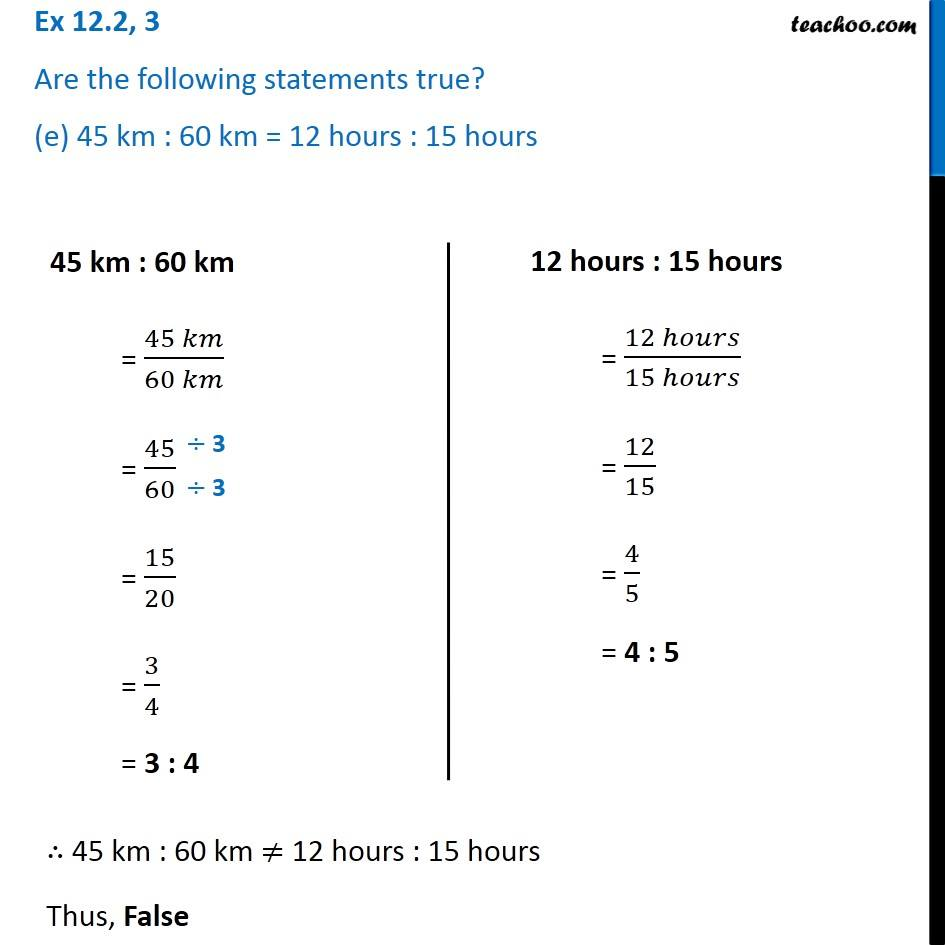Ex 12.2

Chapter 12 Class 6 Ratio and Proportion
Serial order wiseLearn in your speed, with individual attention - Teachoo Maths 1-on-1 Class

### Transcript

Ex 12.2, 3 Are the following statements true? (e) 45 km : 60 km = 12 hours : 15 hours 45 km : 60 km = (45 𝑘𝑚)/(60 𝑘𝑚) = 45/60 = 15/20 = 3/4 = 3 : 4 12 hours : 15 hours = (12 ℎ𝑜𝑢𝑟𝑠)/(15 ℎ𝑜𝑢𝑟𝑠) = 12/15 = 4/5 = 4 : 5 ∴ 45 km : 60 km ≠ 12 hours : 15 hours Thus, False